Home > GC > Chapter 8 > Lesson 8.1.5 > Problem8-55

8-55.
1. Solve for x in each diagram below. Homework Help ✎

1.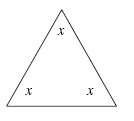2.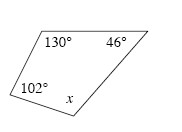3.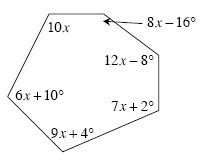4.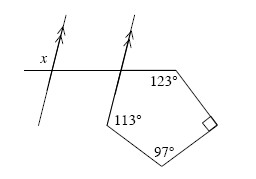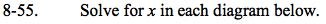Review the Math Notes box in Lesson 8.1.4 for help determining the sum of the interior angles of a polygon.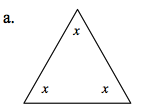x = 60°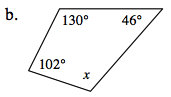x = 82°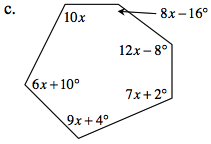10x + (8x − 16) + (12x − 8) + ... = ?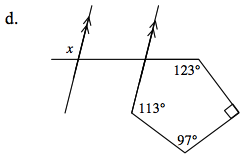How is ∠x and the missing angle in the shape related?
What relationship do they have?